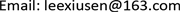SSEMService Science and Management2324-7908Scientific Research Publishing10.12677/SSEM.2018.75018SSEM-26992SSEM20180500000_79436148.pdf经济与管理 基于供应链服务水平优化的双边际化效应分析 Double Marginalization Analyzing Based on Service Level in Supply Chain 秀森1*月君12效义12山东理工大学，数学与统计学院，山东 淄博null060920180705151155© Copyright 2014 by authors and Scientific Research Publishing Inc. 2014This work is licensed under the Creative Commons Attribution International License (CC BY). http://creativecommons.org/licenses/by/4.0/Copyright © 2018 by authors and Hans Publishers Inc.

This work is licensed under the Creative Commons Attribution International License (CC BY).

http://creativecommons.org/licenses/by/4.0/1. 引言

2. 基于成本分担的周期服务水平优化

P——零售价；c——供货价；m——生产成本；r——单位销售剩余由零售商处理的价格( 0 < r < c )；b——单位销售剩余退货给供应商处理的价格( 0 < b < m )；h——单位产品一周期的库存成本(假设 p − c − h > 0 )。

u——供小于求时零售商承担的单位缺货损失(Cost of under-stocking)，假设缺货意味顾客丢失，即 u = p − c − h / 2 ，其中h/2为周期内已销售部分的平均库存成本。

v——供过于求时单位销售剩余成本(Cost of overstocking)，包括单位销售剩余产品(以下简称“剩余”)的库存成本和处理成本。合作模式如下：单位剩余的库存成本h在供销双方之间的分配因子为 β ，零售商分担 β h ，供应商分担 ( 1 − β ) h ；单位剩余的处理成本的分配因子为 γ ，零售商处理剩余时，零售商分担 γ ( c − r ) ，此时零售商承担 v = β h + γ ( c − r ) ；剩余退货给供应商处理时，零售商分担 γ ( m − b ) ，此时零售商承担 v = β h + γ ( m − b ) ， ( 0 ≤ β , γ ≤ 1 ) 。

2.1. 零售商决策的最优服务水平

C S L * = u / ( u + v ) (1)

C S L R * = u u + β h + γ ( c − r ) (2)

C S L V * = u u + β h + γ ( m − b ) (3)

2.2. 整体决策的最优服务水平

C S L SCR * = p − m − h / 2 p − r + h / 2 (4)

C S L SCV * = p − m − h / 2 p − b + h / 2 (5)

2.3. 最优服务水平下的订货策略

π i ( Q i ) = ∫ Q i ∞ Q i U f i ( x i ) d x i + ∫ 0 Q i [ x i U − ( Q i − x i ) V ] f i ( x i ) d x i

Q i * = F i − 1 ( C S L * , μ i , σ i ) (6)

F i − 1 ( ⋅ ) 为 F i ( ⋅ ) 反函数， i = 1 , 2 , ⋯ (证明略)。

3. 基于服务水平优化的风险成本分析

4. 基于服务水平优化的双边际化分析4.1. 零售商处理剩余时双边际化分析

1) 不合作模式

C S L R 0 = p − c − h / 2 p − r + h / 2 (7)

2) 合作模式

3) 算例1

4.2. 供应商处理剩余时的边际化分析

1) 不合作模式和折价退货策略

C S L V 0 = p − c − h / 2 p − c + h / 2 (8)

C S L VD 0 = p − c − h / 2 p − d + h / 2 。因为d < c，所以 C S L VD 0 < C S L V 0 ，即“折价退货策略”可以抑制零售商盲目提高服务水平，从而减弱这种盲目决策对于整体的伤害。

2) 合作模式

3) 算例2

5. 结束语